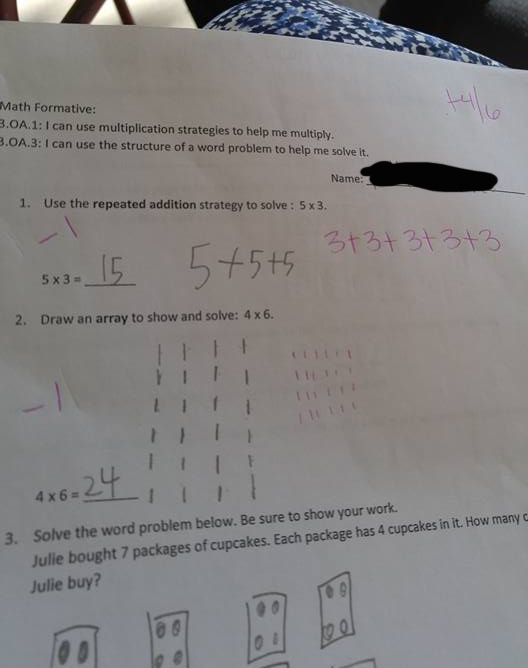# A student's "5+5+5=15" answer was marked as incorrect. Why?

Published: 28/04/2016The question posed by the teacher was "what is 5x3?". The child answered "15", as given by the calculation "5+5+5". However, the teacher was expecting the answer 3+3+3+3+3=15, and hence marked the child’s answer as incorrect. The question is part of the common core mathematical knowledge required of every third grader in America. The child is expected to understand and see 5x3 as five groups of three and not three groups of five. The child’s answer to the next question was also marked as incorrect where he saw six groups of four instead of four groups of six.Read more about Commutativity: In mathematics, a binary operation is commutative if changing the order of the operands does not change the result. It is a fundamental property of many binary operations, and many mathematical proofs depend on it. Most familiar as the name of the property that says "3 + 4 = 4 + 3" or "2 × 5 = 5 × 2", the property can also be used in more advanced settings. See: https://en.wikipedia.org/wiki/Commutative_property

The image was posted on the popular image sharing website Reddit and shared over the internet. One of most upvoted comments was “So now you just took a kid who was doing well at math, confused them, and turned them off of it with your continuous nit picking of shit that doesn't matter.”. Asking around our own office, the DNA testing staff are puzzled by the requirements: for us it is more important to see that actual result from the equation 5x3 is the same as 3x5 instead of some ridiculous meaning assigned to order of digits, with no foundation in math itself.